# Questions on gravitation for JEE Main/Advanced/AP physics

In this page we have Questions on gravitation for JEE Main/Advanced/AP physics . Hope you like them and do not forget to like , social share and comment at the end of the page.
Question 1:
Which of the following is true for Uniform Solid sphere  of  Mass M and radius b
a) for r > b,Gravitational field decreases as r is increased
b) for r< b, Gravitational field increased as r is increased
c) Gravitational Field at r=0 is equal to 0
d) Gravitational field at the r=b is GM/b2
Question 2:
Let  P denotes the Gravitational potential and F denotes the Gravitational Field
What all relation are true for a uniform spherical shell
a) P≠0 inside the shell and it is constant inside the shell
b) E≠0 inside the shell and it is constant inside the shell
c) E=0 at all the point inside the shell
d) none of these
Question 3:
3 A thin rod of length L and mass M is placed along x axis.One end of rod is placed at origin and other end is at (L,0).
Let i and j are the unit vector across x and y axis
Find the Gravitational field at Point P( a,0)  where a > L
a)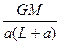i
b)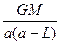i
c)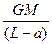i
d ) None of these
Question 4:
Find the gravitational potential  at point P .We take gravitational potential to be zero at infinity
a)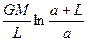b)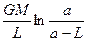c)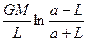d)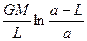Question 5:
Three particles A,B,C each of mass m are placed at the corner of the  equilateral triangle of side a. Find the potential energy of the system
A,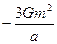B)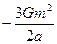C)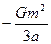D) None of these
Question 6:
Find the Gravitational Potential at point A
a)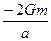b)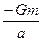c)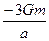d) None of these
Question 7:
Find the work done on this system if the side of the triangle is changed from a to 3a
a)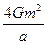b)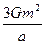c)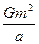d)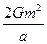Question 8:
Three concentric shells A,B ,C of uniform density have masses M1 ,M2 and M3 respectively. There radii are R1,R2 and R3  respectively.
R3 > R2 > R1.
A particle of mass m is placed at placed at a distance d
Match the column
Column A  ( it tells us about the location)
P)  R1 < d  < R2
Q)  d < R1
R) R1  < R2 < d < R3
S)  R1  < R2  < R3 < d
Column B ( it tells us about the gravitational force experienced)
D)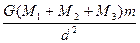E)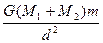F)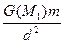G) 0
Question 9:
A cosmic body A  of mass m moves to the sun  with velocity v0 ( when far from sun) and aiming parameter d  the arm of the velocity v0 relative to the center of the sun.
1) Find the angular momentum of the body about the sun at the starting point
2) Find the minimum distance which this body will get to the sun
3) Find the velocity at minimum separation
4) Find the total energy of the system at the minimum distance
Mass of the sun =Ms
Question 10:
A planet of mass M moves along a ellipse around the sun  so that its maximum and minimum distance from the sun are equal to a  and b respectively
a) Find the velocities at the minimum and maximum distance
b) Find the Total energy of the system
c) Find the angular momentum of the planet relative to the center of the sun
d) At a point P when at a distance r0 from the sun,the angle between velocity vector and radius vector is θ. Find the velocity at the point P
e) find the ecentricty and semi major axis of the ellipse
Mass of the sun =m
Question 11:
A hole were drilled completely  through  the earth  along a diameter.A particle of mass m is released into the hole from the surface of the earth
a) find the force acting on the mass m at distance r from the center
b) Show that the motion will be simple harmonic  and Find out the time period of it
Let M be the mass of the Earth
R be the radii of the Earth
We assume that density is Uniform
Question 12:
Two satellite M and N are revolving around the earth in coplanar concentric orbits.
The Time period of the satellite are 2 h and 16 H respectively. The radius of the orbit of the satellite N is 3.2X 104 Km.The satellite are moving in opposite direction.
At t=0, the position of the satellite is shown in below figure
Find out the following things
1) The time period of the satellite  M
2) Orbital velocity of both the satellite
3)  Find the angular velocity of satellite N as observed  by M at t=8 hour
Question 13:
A satellite of mass m  is orbiting the earth in a circular  orbit of radius R.
Statement I: Angular Momentum of the satellite varies as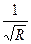Statement II: Linear momentum of the satellite varies as 1/R
Statement III: Kinetic energy varies as 1/R
Statement IV:  Frequency of revolution varies as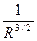Which of the following  is correct
a) All the statements are correct
b) Statement I,II,III are correct only
c) Statement II,IV are correct only
d) Statement I,II,IV are correct only

Solutions